library(patchwork)

While quite complex compositions can be achieved using +, |, and /, it may be necessary to take even more control over the layout. All of this can be controlled using the plot_layout() function along with a couple of special placeholder objects. We’ll use our well-known mtcars plots to show the different options.

library(ggplot2)
p1 <- ggplot(mtcars) +
geom_point(aes(mpg, disp)) +
ggtitle('Plot 1')

p2 <- ggplot(mtcars) +
geom_boxplot(aes(gear, disp, group = gear)) +
ggtitle('Plot 2')

p3 <- ggplot(mtcars) +
geom_point(aes(hp, wt, colour = mpg)) +
ggtitle('Plot 3')

p4 <- ggplot(mtcars) +
geom_bar(aes(gear)) +
facet_wrap(~cyl) +
ggtitle('Plot 4')

Sometimes all that is needed is to have an empty area in between plots. This can be done by adding a plot_spacer(). It will occupy a grid cell in the same way a plot without any outer elements (titles, ticks, strips, etc.):

p1 + plot_spacer() + p2 + plot_spacer() + p3 + plot_spacer()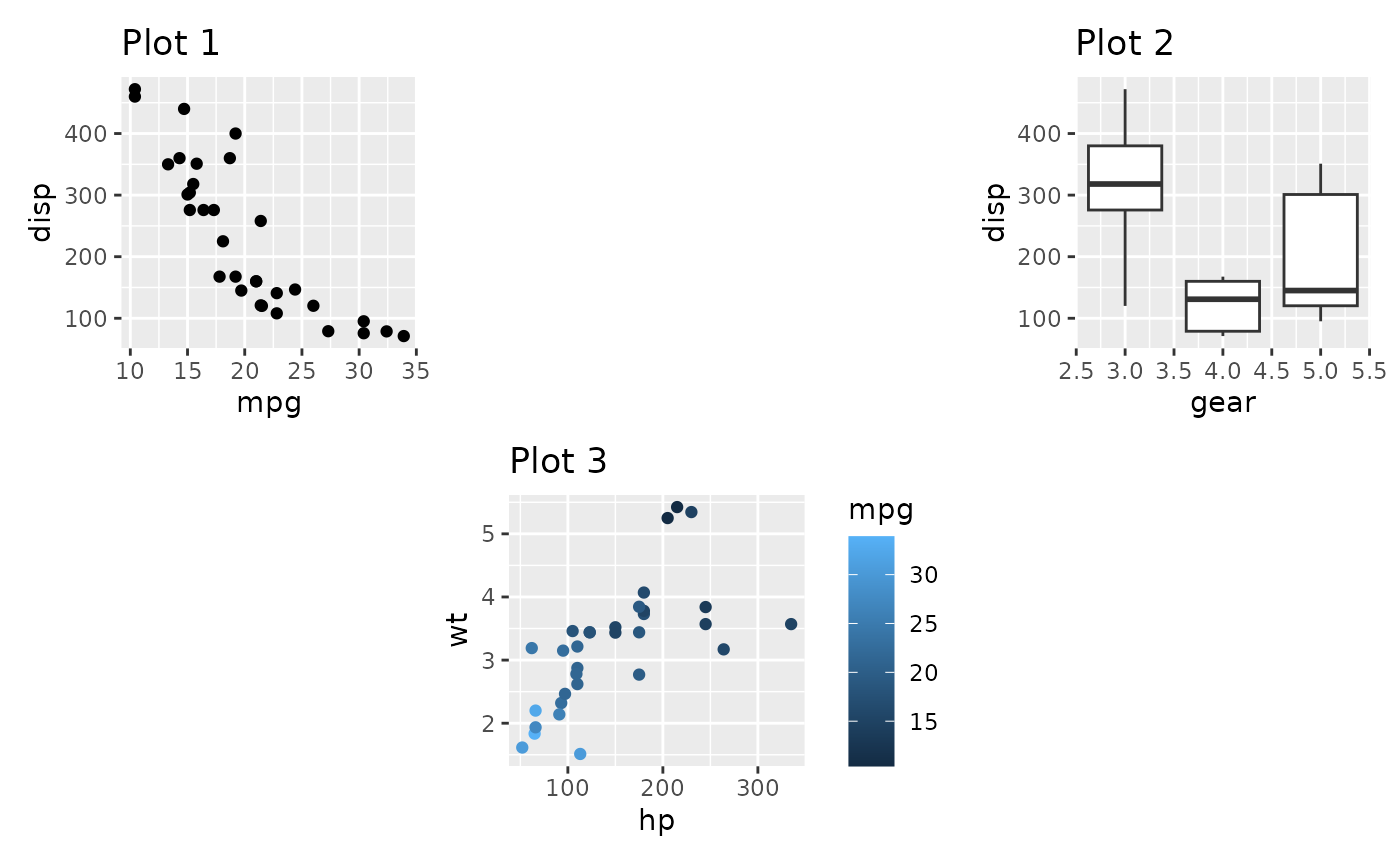It is important to understand that the added area only corresponds to the size of a plot panel. This means that spacers in separate nesting levels may have different dimensions:

(p1 + plot_spacer() + p2) / (plot_spacer() + p3 + plot_spacer())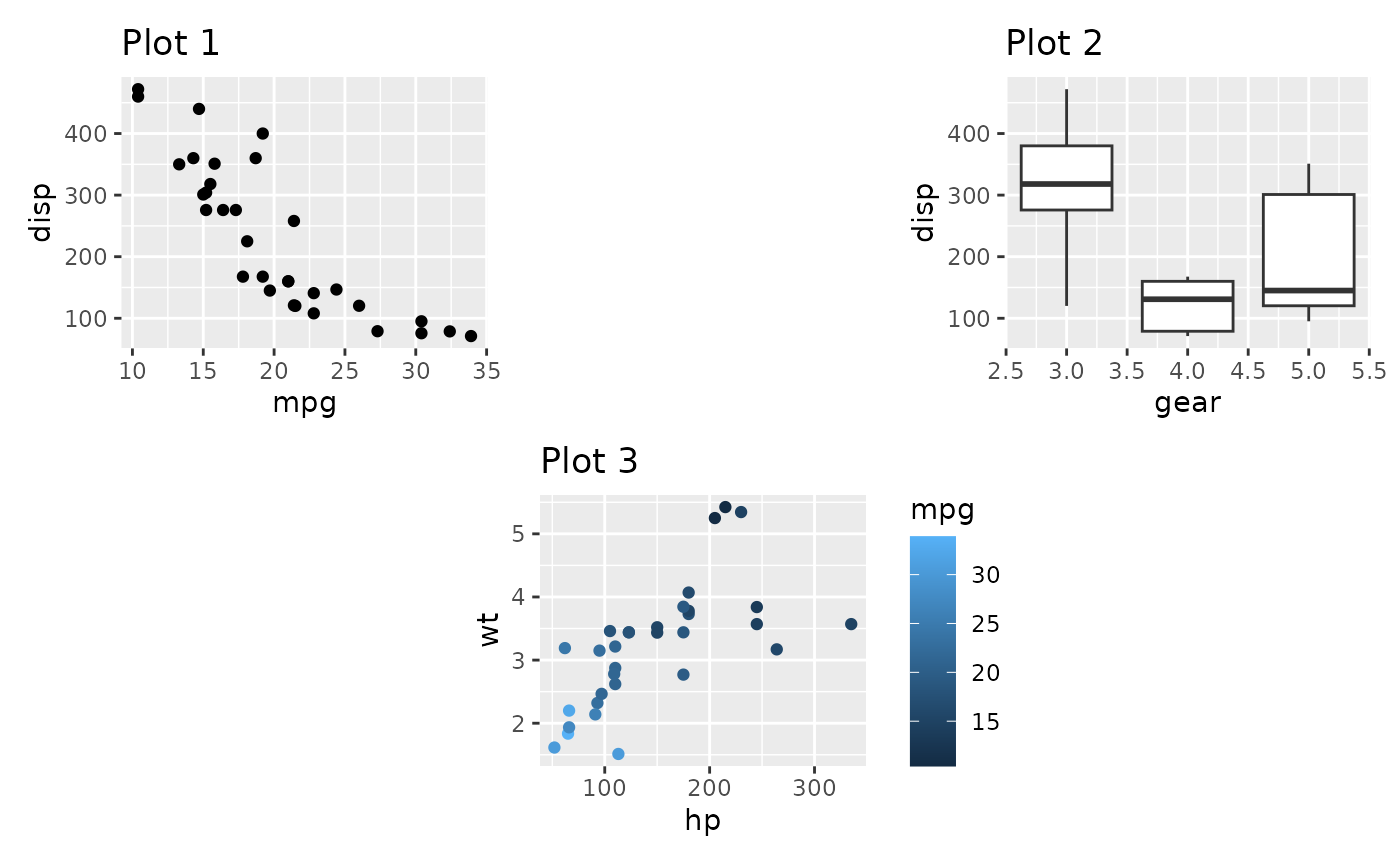## Controlling the grid

If nothing is given, patchwork will try to make a grid as square as possible, erring to the side of a horizontal grid if a square is not possible (it uses the same heuristic as facet_wrap() in ggplot2). Further, each column and row in the grid will take up the same space. Both of these can be controlled with plot_layout()

p1 + p2 + p3 + p4 +
plot_layout(ncol = 3)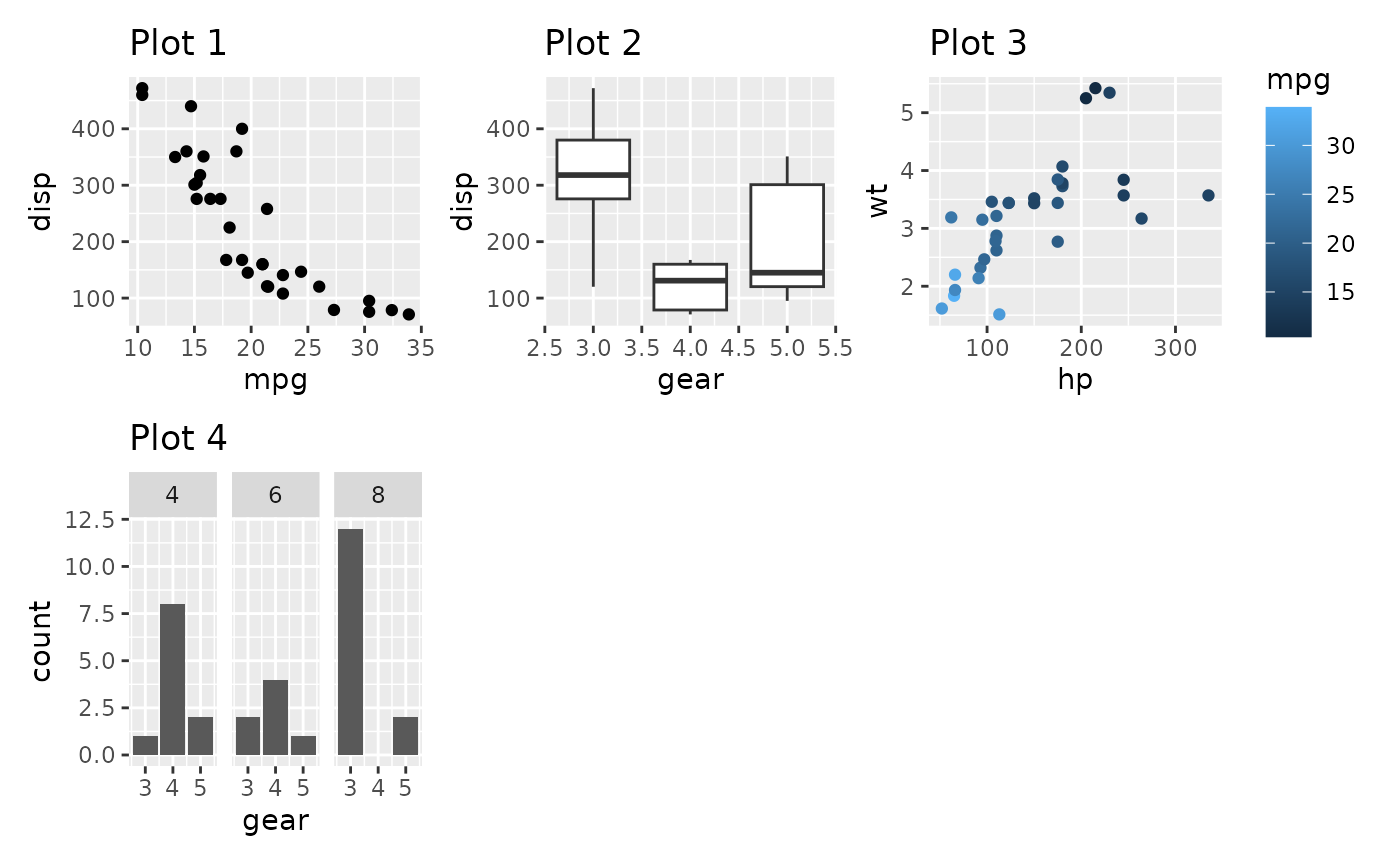p1 + p2 + p3 + p4 +
plot_layout(widths = c(2, 1))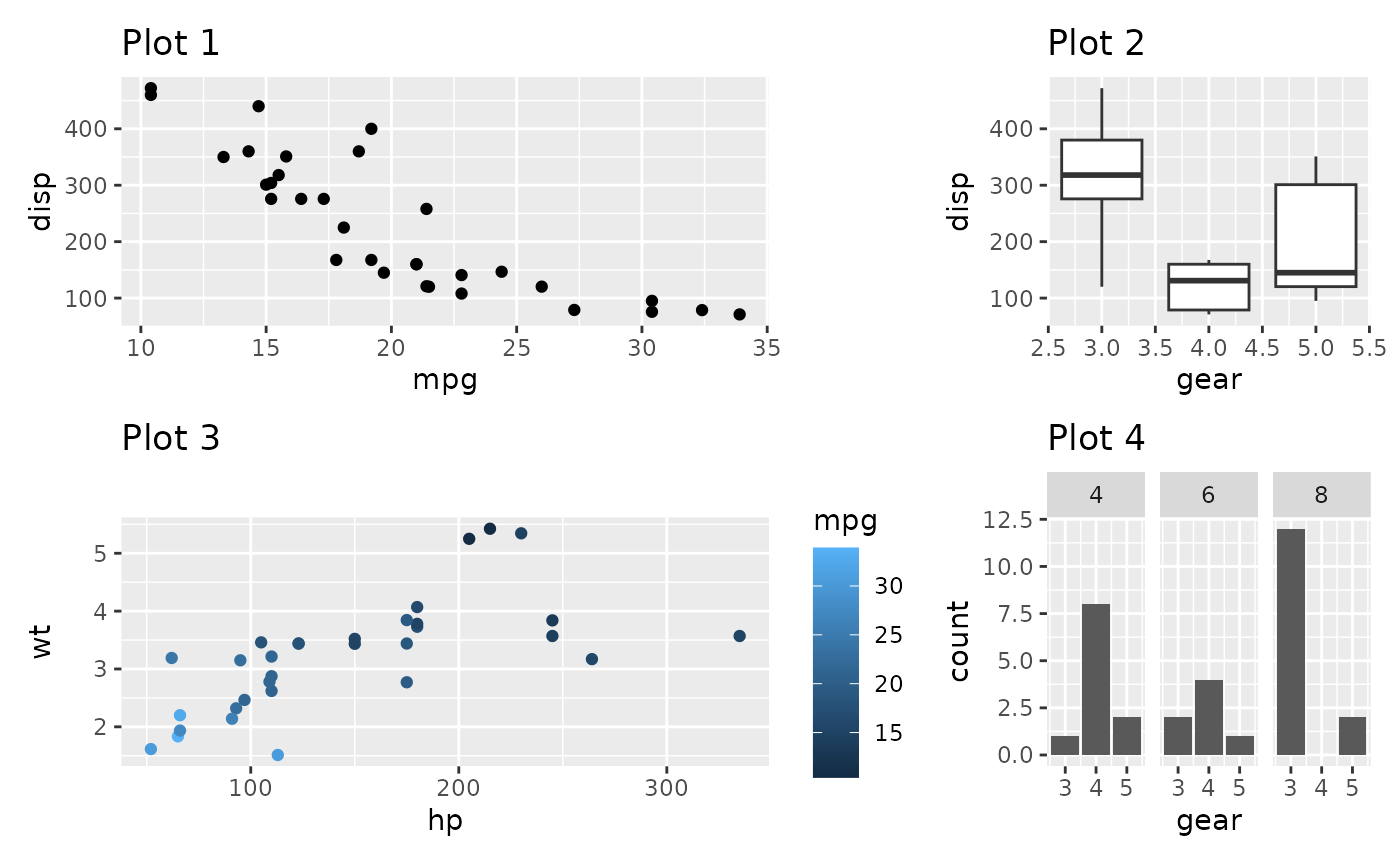When grid sizes are given as a numeric, it will define the relative sizing of the panels. In the above, the panel area of the first column is twice that of the second column. It is also possible to supply a unit vector instead:

p1 + p2 + p3 + p4 +
plot_layout(widths = c(2, 1), heights = unit(c(5, 1), c('cm', 'null')))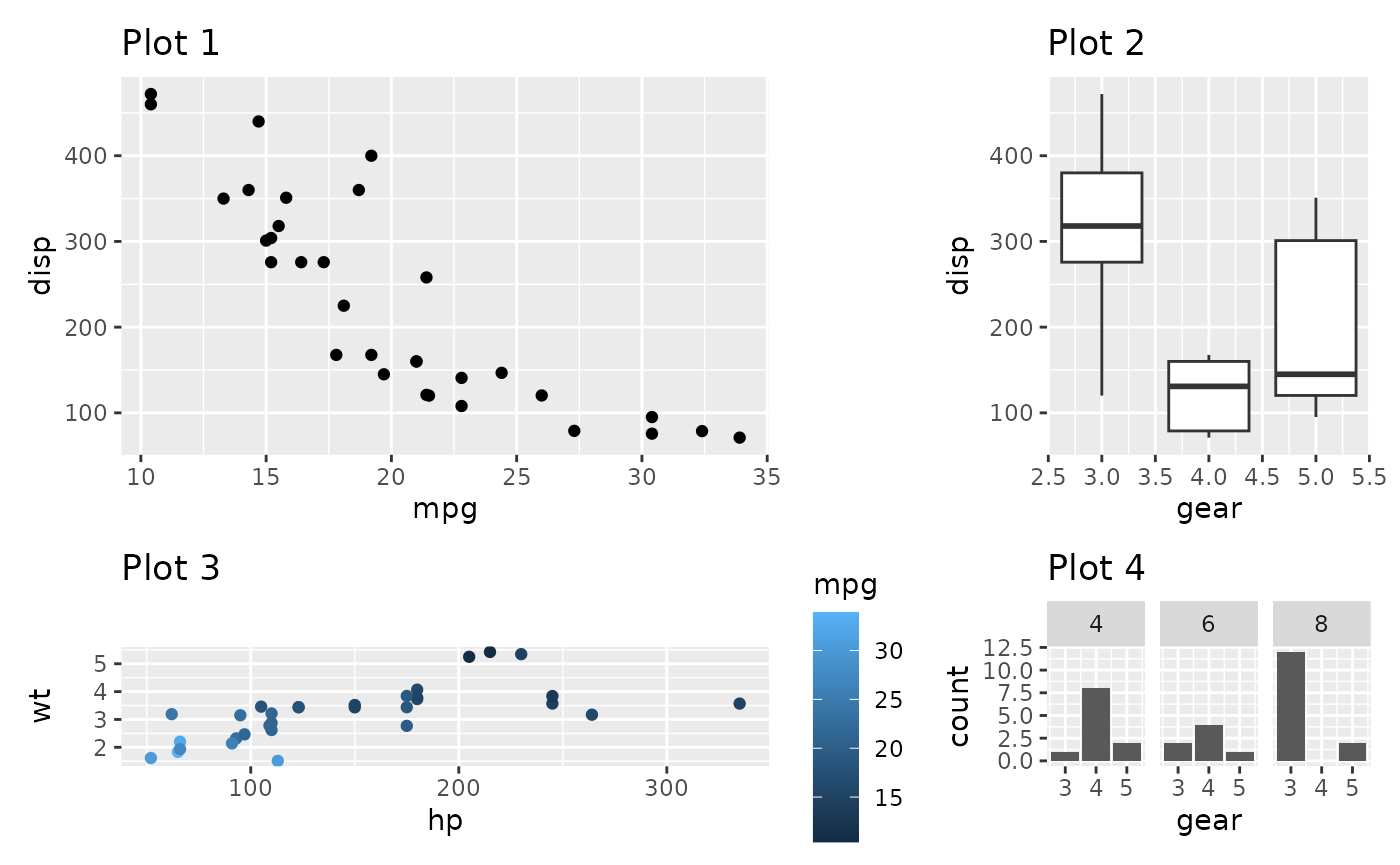In the last example the first row will always occupy 5cm, while the second will expand to fill the remaining area.

It is important to remember that sizing only affects the plotting region (panel area). If a plot has, e.g., very wide y-axis text it will not be penalized and get a smaller overall plotting region.

## Moving beyond the grid

Earlier, when we’ve wanted to create non-grid compositions, we’ve used nesting. While this is often enough, you end up losing the alignment between subplots from different nested areas. An alternative is to define a layout design to fill the plots into. Such a design can be defined in two different ways. The easiest is to use a textual representation:

layout <- "
##BBBB
AACCDD
##CCDD
"
p1 + p2 + p3 + p4 +
plot_layout(design = layout)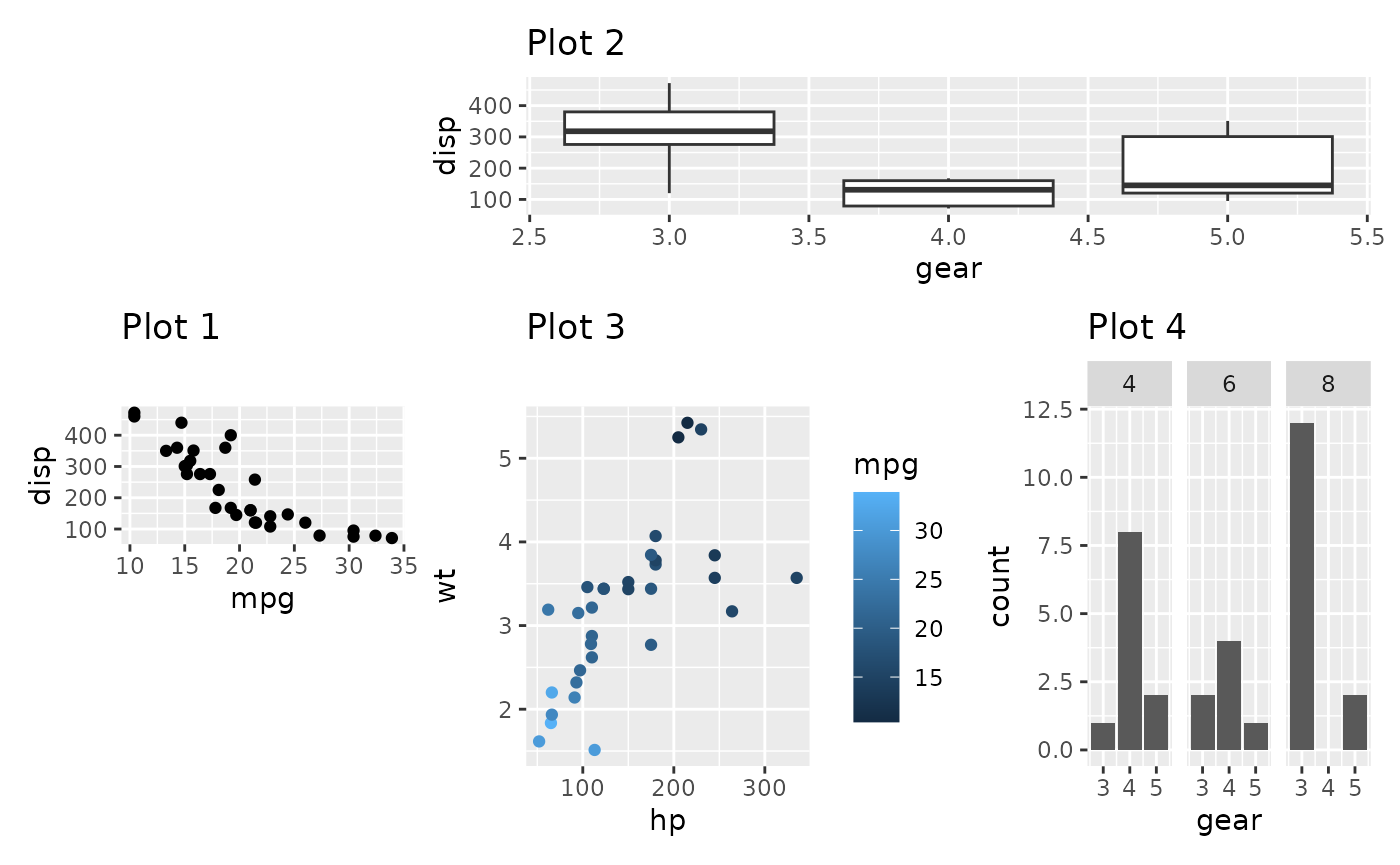When using the textual representation it is your responsibility to make sure that each area is rectangular. The only exception is # which denotes empty areas and can thus be of any shape.

A more programmatic approach is to build up the layout using the area() constructor. It is a bit more verbose but easier to program with. Further, this allows you to overlay plots.

layout <- c(
area(t = 2, l = 1, b = 5, r = 4),
area(t = 1, l = 3, b = 3, r = 5)
)
p1 + p2 +
plot_layout(design = layout)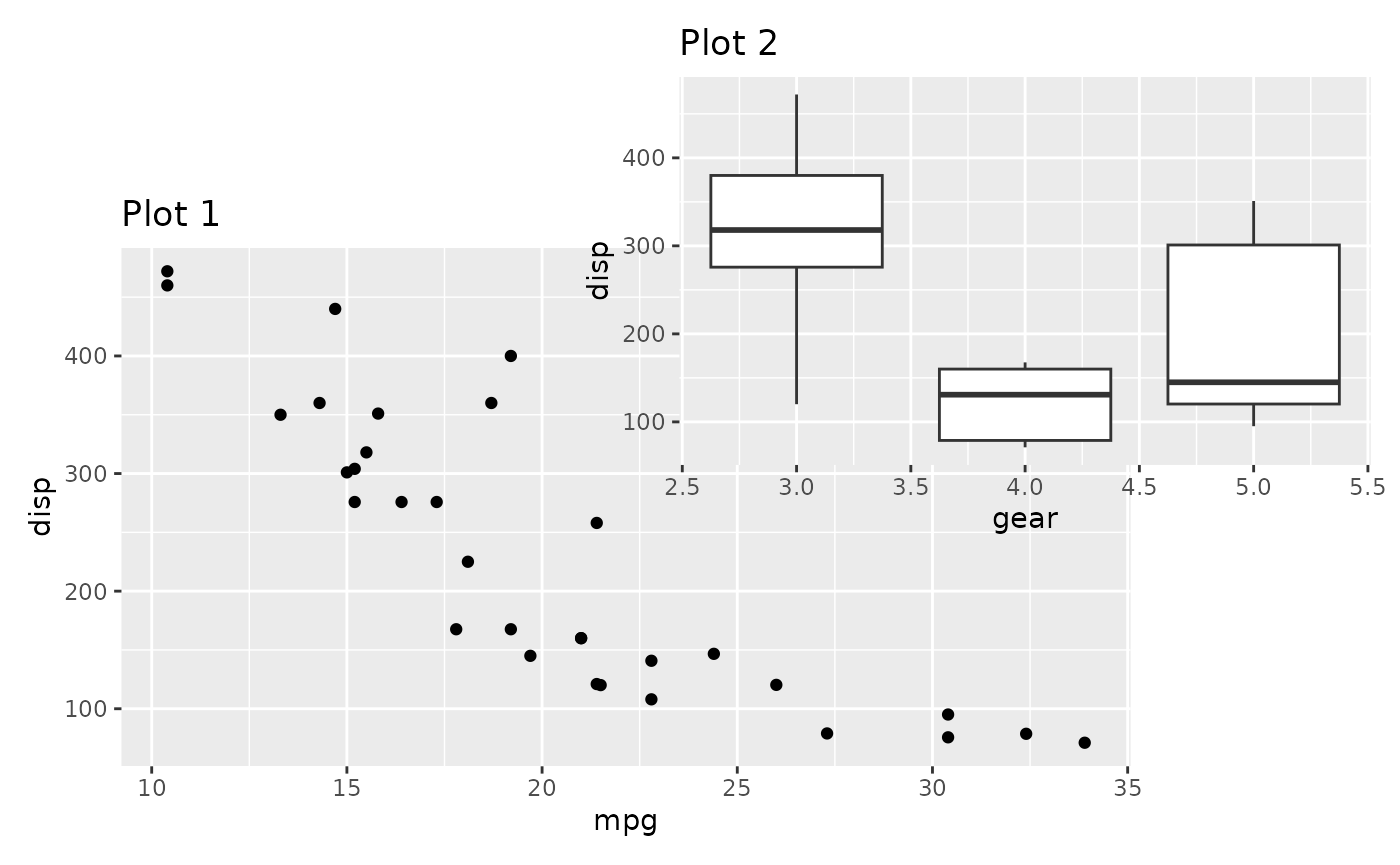The design specification can of course also be used with widths and heights to specify the size of the columns and rows in the design.

A small additional feature of the design argument exists if used in conjunction with wrap_plots() function (See the Plot Assembly guide). If the design is given as a textual representation, you can name the plots to match them to the different areas, instead of letting them be filled in in the order they appear:

layout <- '
A#B
#C#
D#E
'
wrap_plots(D = p1, C = p2, B = p3, design = layout)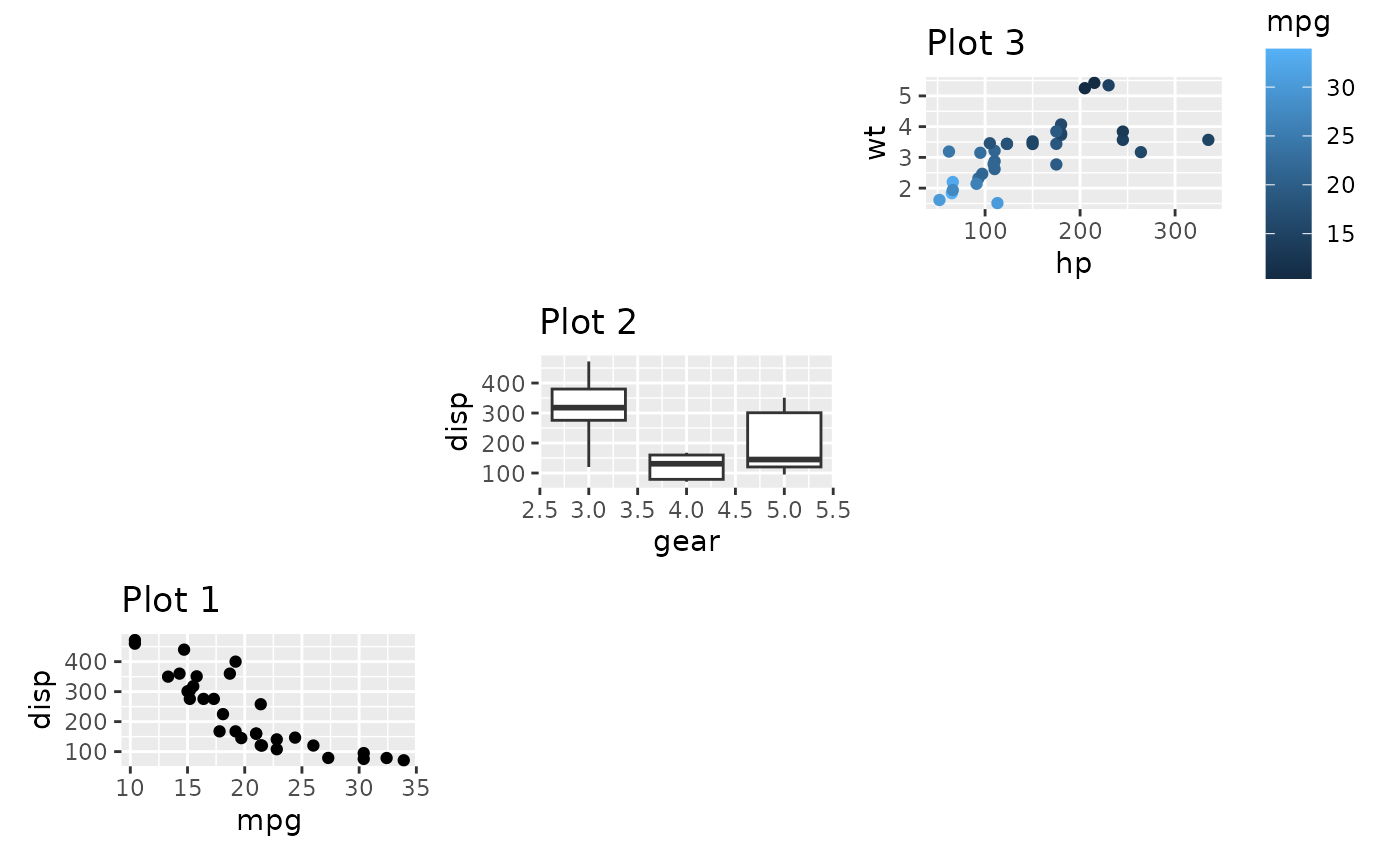## Fixed aspect plots

A special case when it comes to assembling plots are when dealing with fixed aspect plots, such as those created with coord_fixed(), coord_polar(), and coord_sf(). It is not possible to simultaneously assign even dimensions and align fixed aspect plots. The default value for the widths and heights arguments in plot_layout() is NA, which is treated specially. In general it will behave just as 1null (i.e. expand to fill available space but share evenly with other 1null panels), but if the row/column is occupied by a fixed aspect plot it will expand and contract in order to keep the aspect of the plot and may thus not have the same dimension as grid width/heights containing plots without fixed aspect plots. If you need to mix this behavior with fixed dimensions you can use the special value of -1null which behaves the same as NA (unit vectors doesn’t allow NA values). width = unit(c(1, 3, -1), c("null", "cm", "null") would specify one column that fills out available space, one column fixed to 3 cm, and one column that expands to match a fixed aspect ratio if needed or otherwise takes the same size as the first column.

p_fixed <- ggplot(mtcars) +
geom_point(aes(hp, disp)) +
ggtitle('Plot F') +
coord_fixed()
p_fixed + p1 + p2 + p3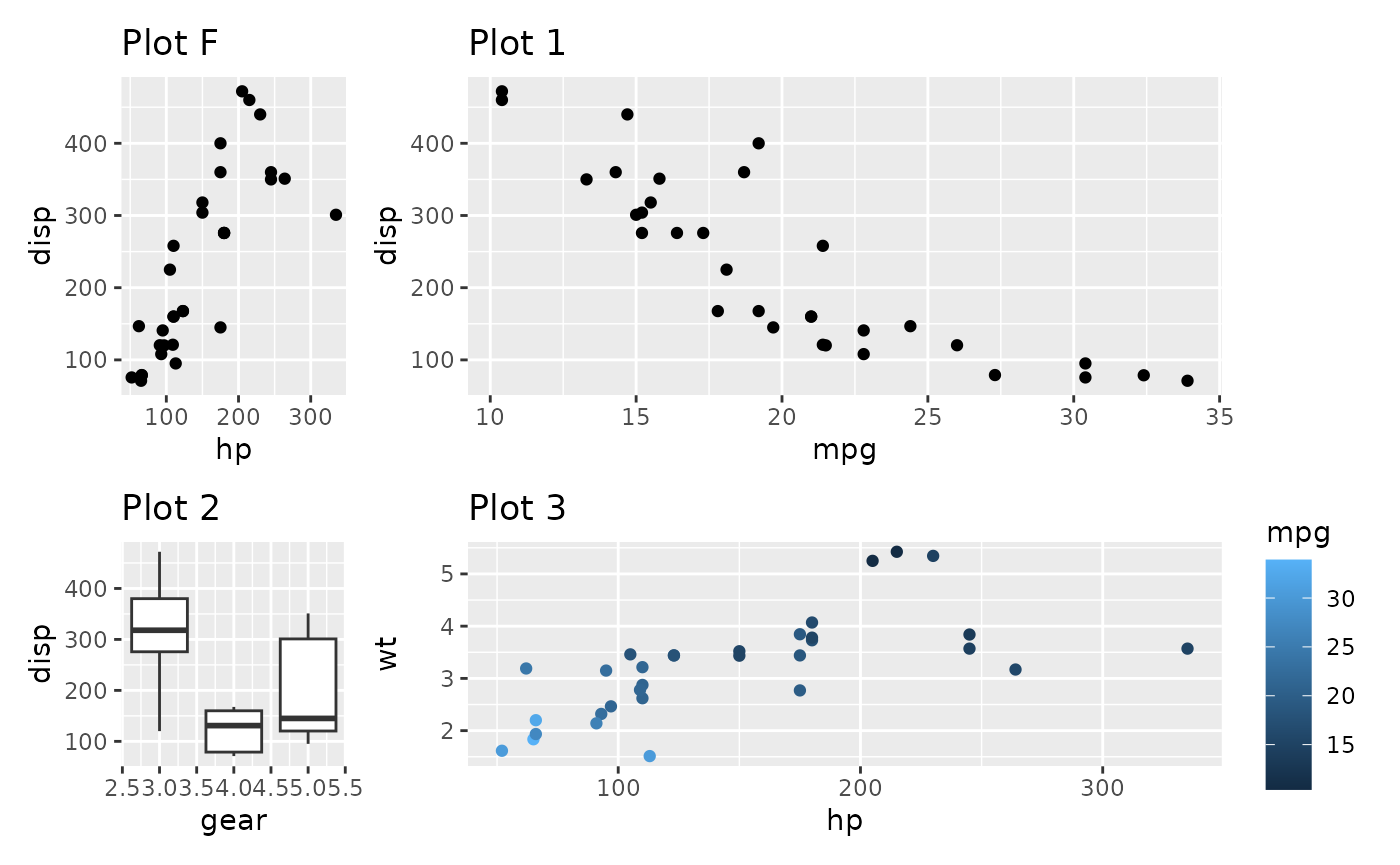Contrast this with setting the widths to a non-NA value:

p_fixed + p1 + p2 + p3 + plot_layout(widths = 1)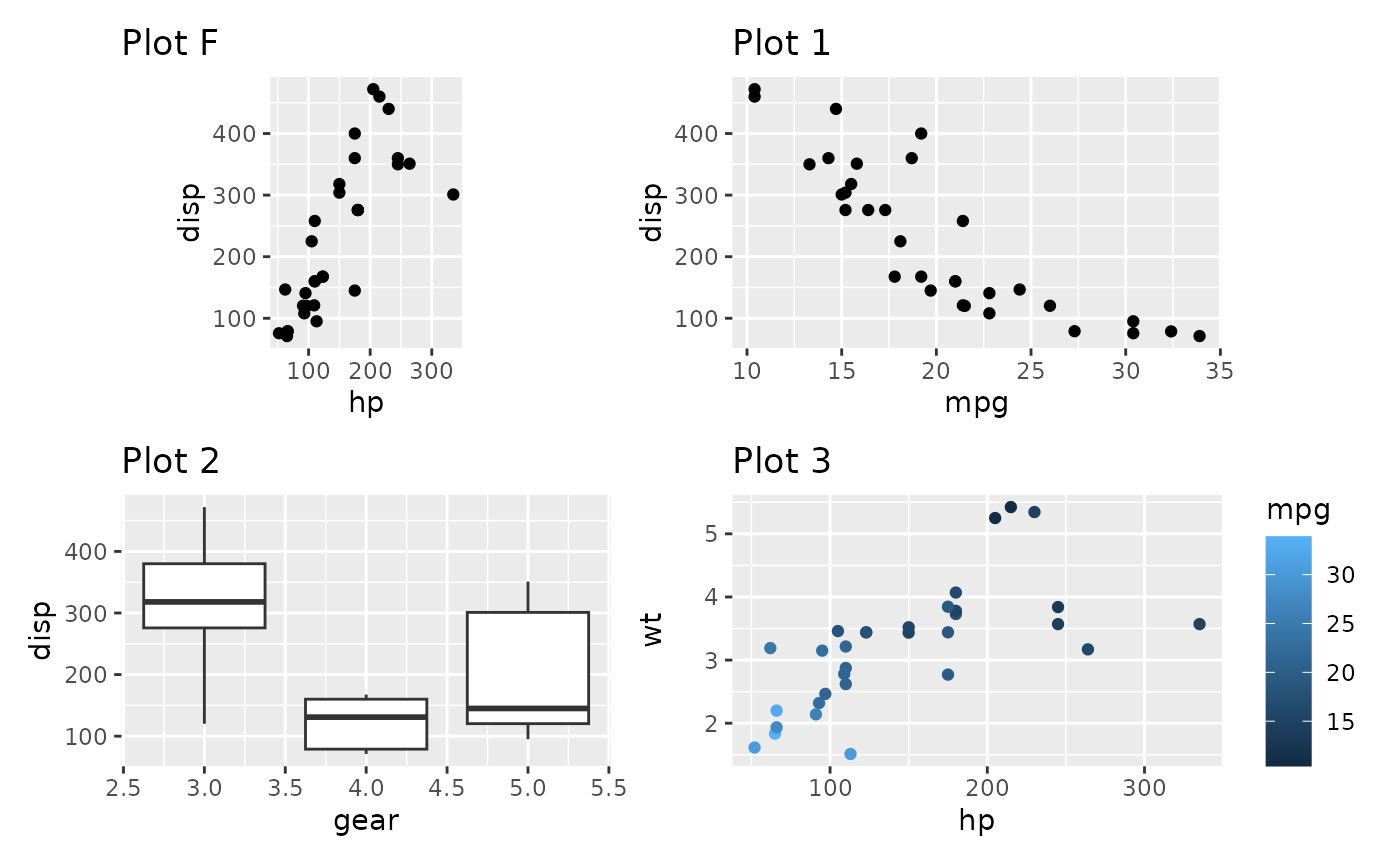As you can see, the fixed aspect plot keeps its aspect ratio, but loses the axis alignment in one of the directions. Which solution is needed is probably dependent on the specific use case. The default optimizes the use of space.

There are some restrictions to the space optimization. The fixed aspect plot cannot take up multiple rows or columns, and if one fixed aspect plot conflicts with another one, one of them will end up not using the full space.

## Avoiding alignment

Patchwork is designed to do it’s utmost to align the plotting areas, and while this is generally sensible in order to create a calm and good looking layout it sometimes gets in the way of creating what you want. Below we will see two such cases. In both situation the answer is the wrap_elements() function.

### Huge axis text causing white space.

Sometimes one of the plots has much longer axis text or axis labels than the other:

p2mod <- p2 + labs(x = "This is such a long\nand important label that\nit has to span many lines")
p1 | p2mod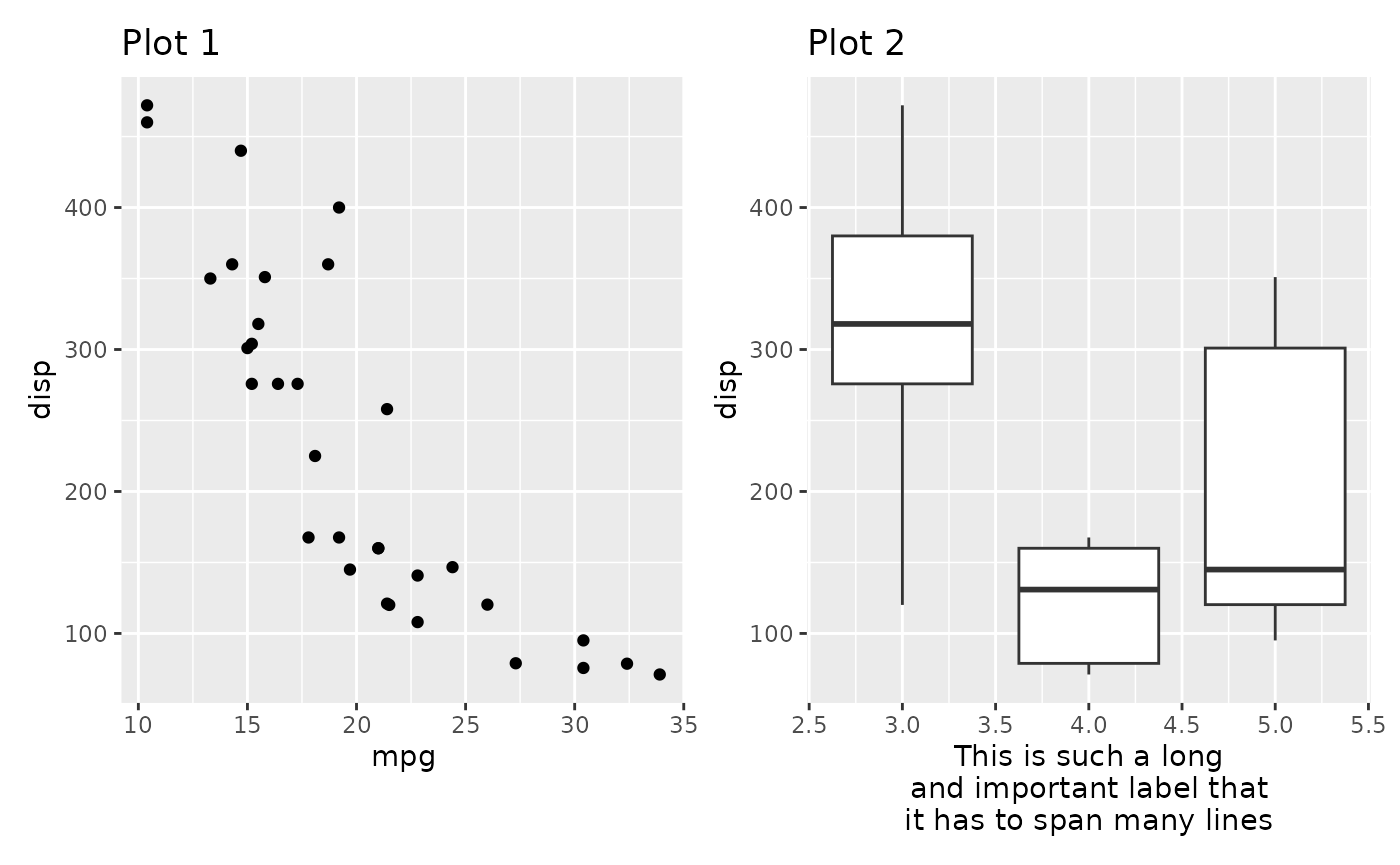Depending on what you prefer you might want the leftmost plot to fill out as much room as possible instead of being aligned to the rightmost panel. Putting a ggplot or a patchwork inside wrap_elements() removes any alignment from the plot.

wrap_elements(full = p1) | p2mod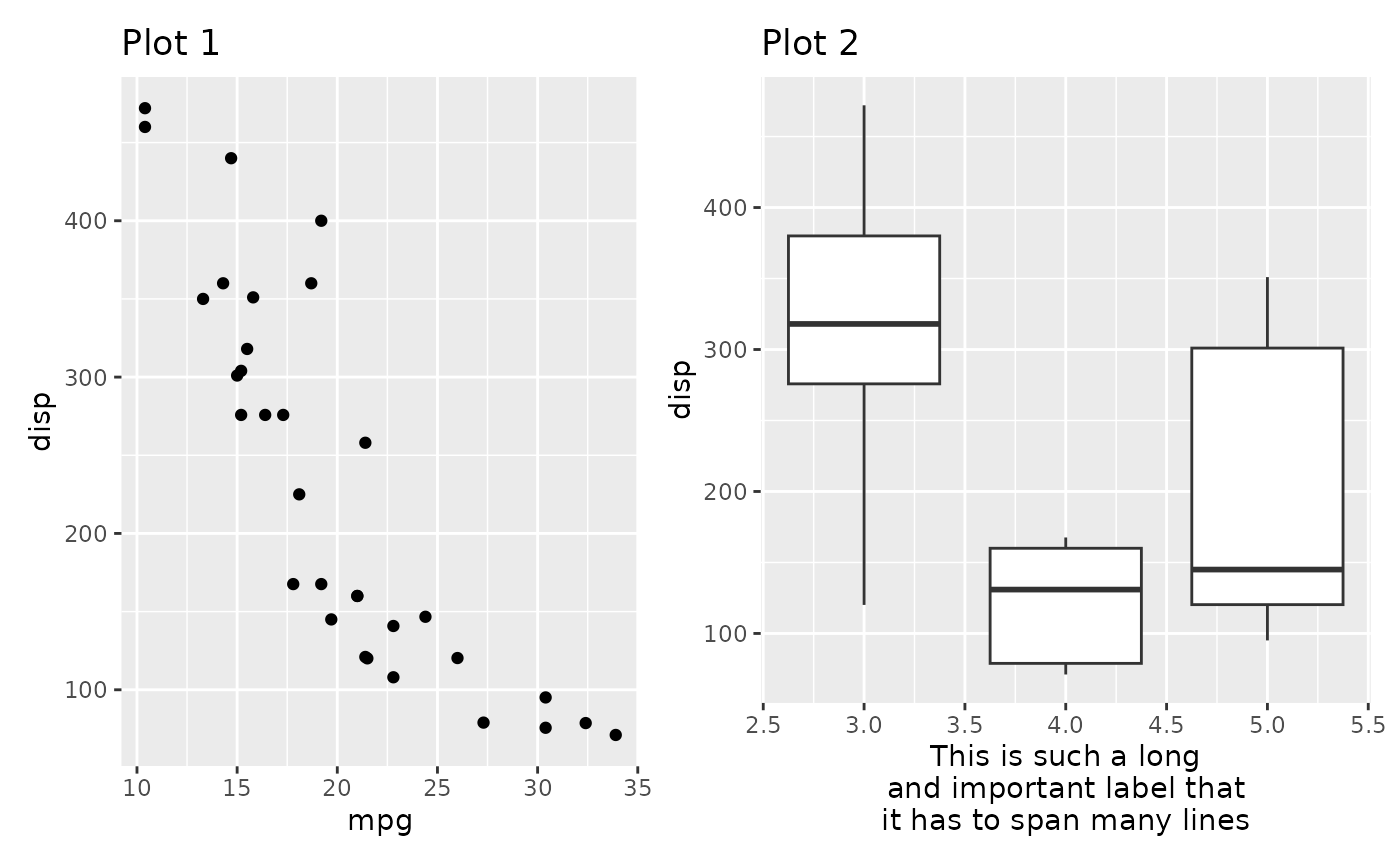### Designs don’t keep an even width

When creating a complex layout using a design you may create setups where plots spans the edge of other plots and be surprised how that affects the width of the panels in the design:

design <- "#AAAA#
#AAAA#
BBCCDD
EEFFGG"
p3 + p2 + p1 + p4 + p4 + p1 + p2 +
plot_layout(design = design)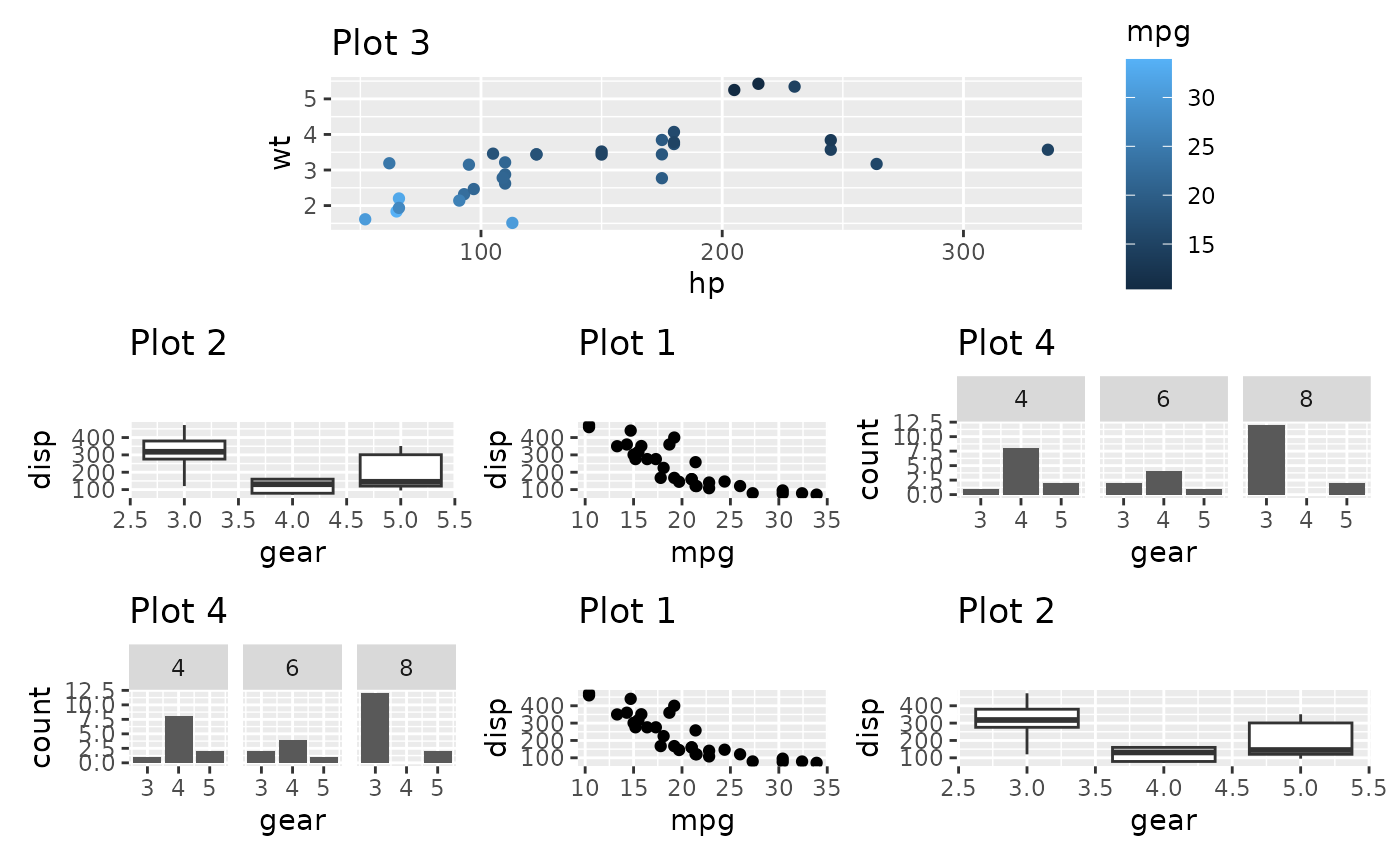In the above we see that the 3 bottom columns all have different widths despite being given the same amount of space in the design matrix. The reason for this is that the top plot has a y-axis to the left and a guide to the right, and those fall in between the two columns that make up the 1st and 3rd column in the bottom. Subsequently those plots are expanded to fill out the space. Once again wrap_elements() will save us from this:

wrap_elements(full = p3) + p2 + p1 + p4 + p4 + p1 + p2 +
plot_layout(design = design)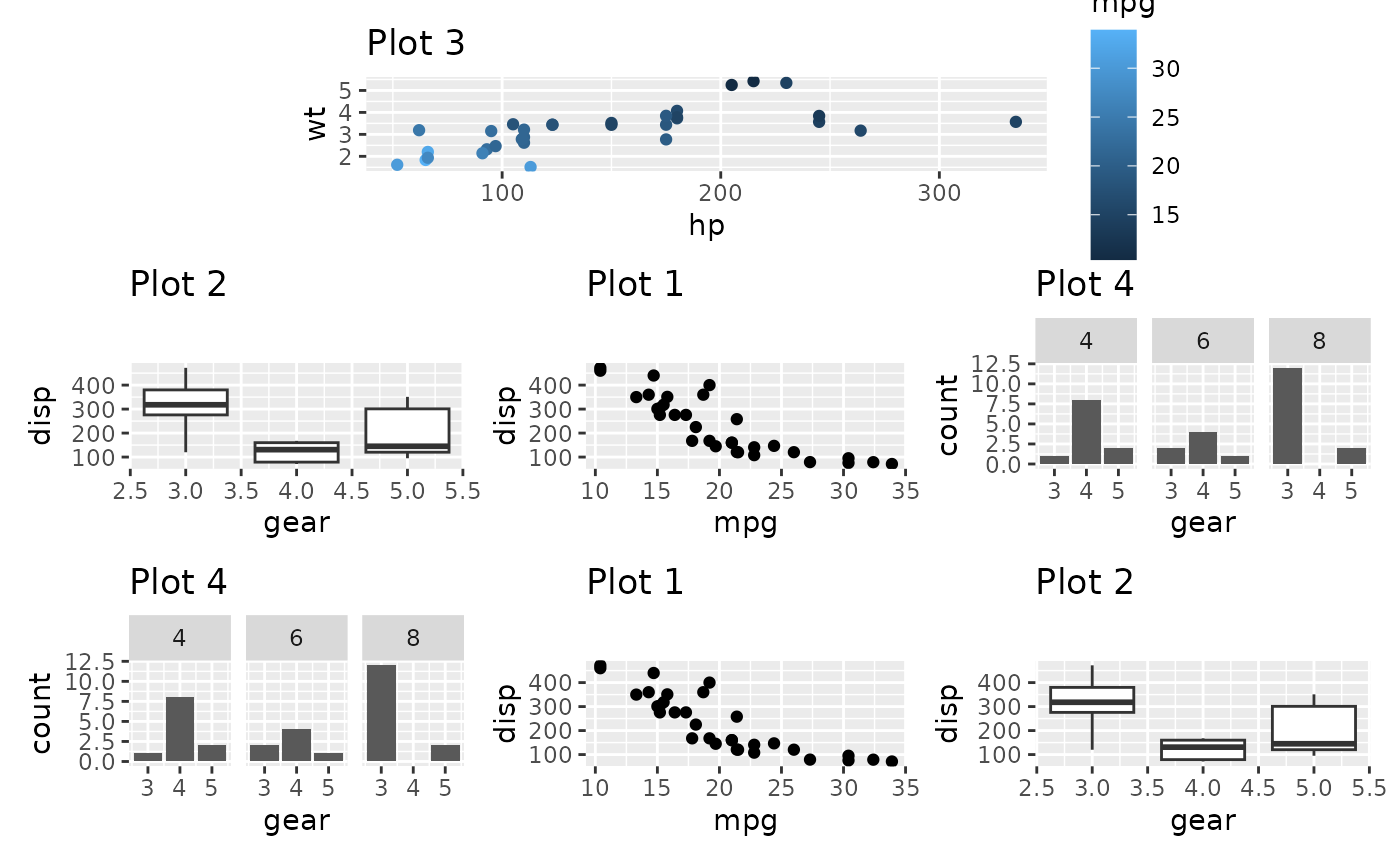## Insets

An alternative to placing plots in a grid is to place a plot as an inset in another plot. As we saw above, this is achievable with a setting up a layout with multiple overlapping area() specifications. However, this approach still uses an underlying grid, which may be constraining. Another approach is to use the inset_element() function which marks a plot or graphic object to be placed as an inset on the previous plot. It will thus not take up a slot in the provided layout, but share the slot with the previous plot. inset_element() allows you to freely position your inset relative to either the panel, plot, or full area of the previous plot, by specifying the location of the left, bottom, right, and top edge of the inset.

p1 + inset_element(p2, left = 0.6, bottom = 0.6, right = 1, top = 1)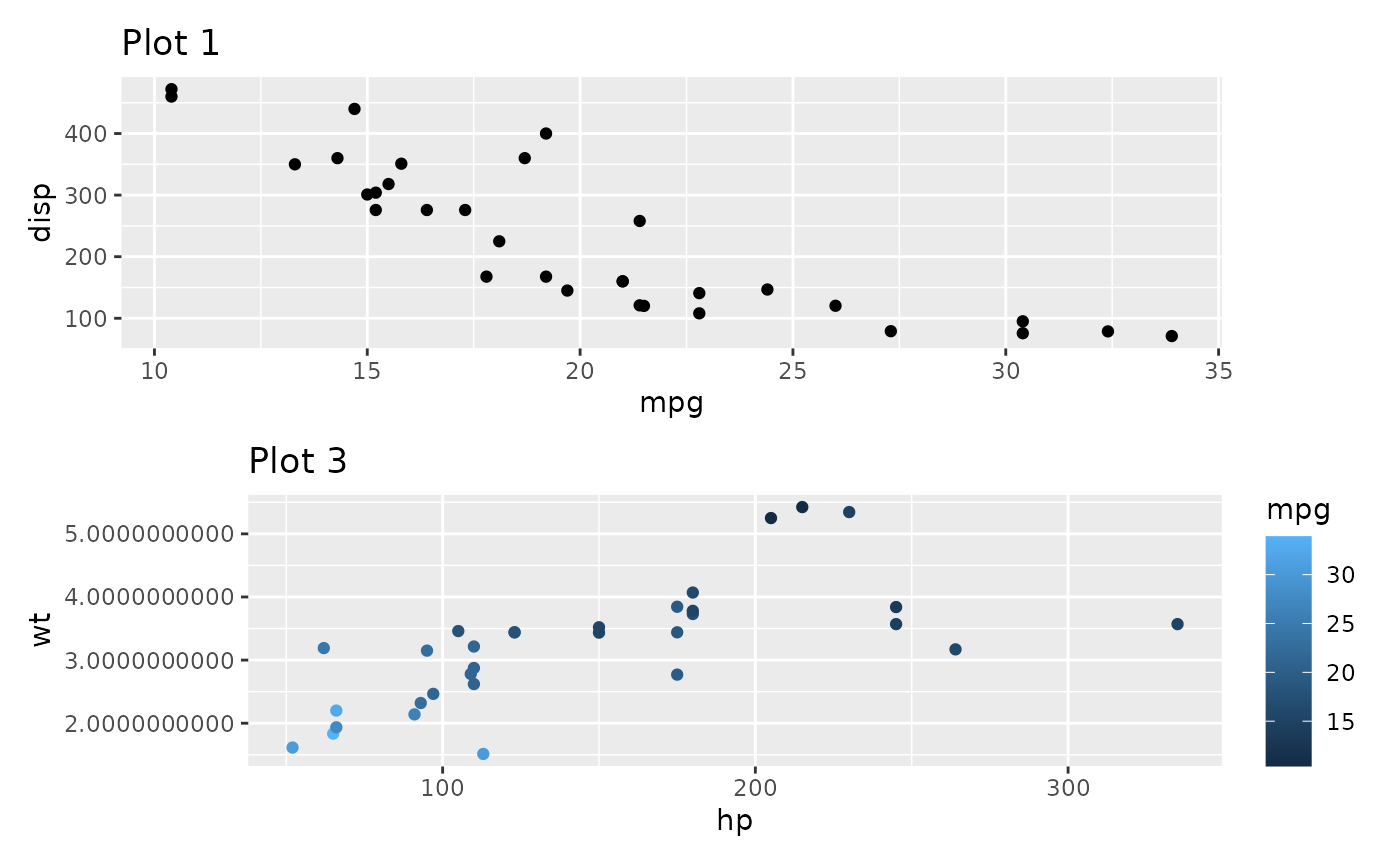p1 + inset_element(p2, left = 0, bottom = 0.6, right = 0.4, top = 1, align_to = 'full')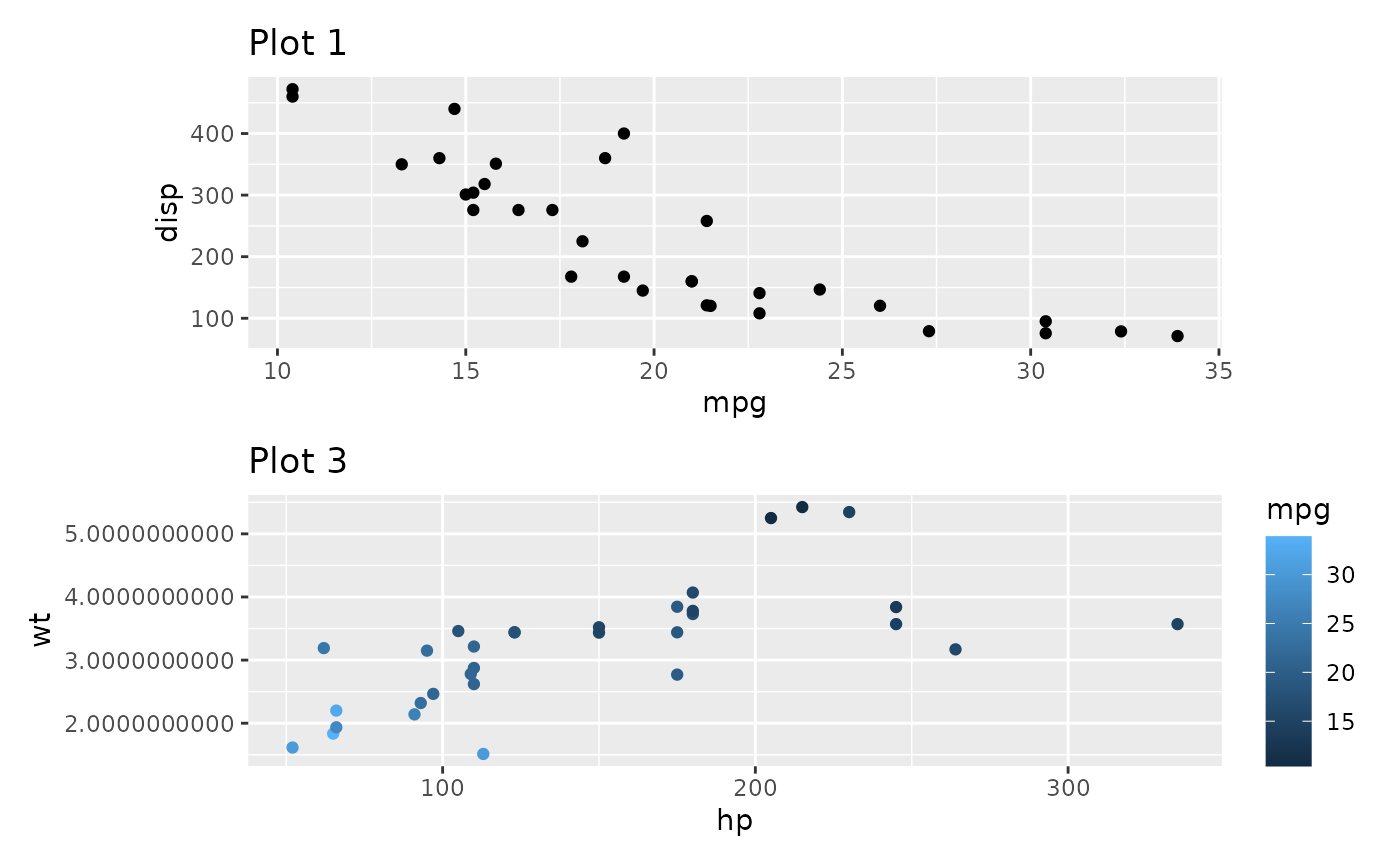The location can be specified either as numerics like above or as grid units. If specified as numerics they will be converted to 'npc' units which goes from 0 to 1 in the specified area. As an example of this we will place an inset exactly 1 cm from the panel border in the code below:

p1 + inset_element(
p2,
left = 0.5,
bottom = 0.5,
right = unit(1, 'npc') - unit(1, 'cm'),
top = unit(1, 'npc') - unit(1, 'cm')
)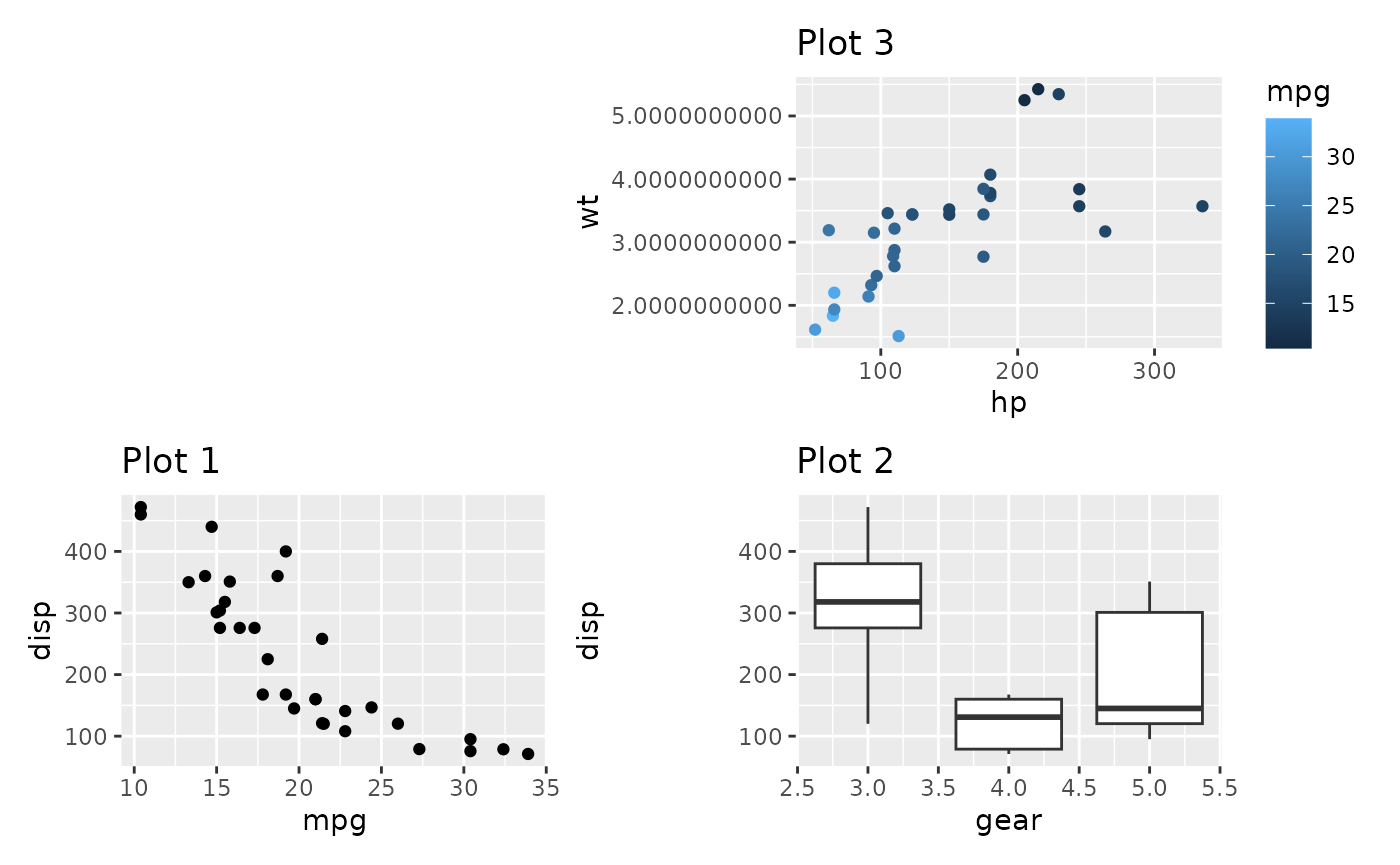Further, inset_element() allows you to place the inset below the previous plot, should you choose to, and controlling clipping and tagging in the same way as wrap_elements().

p3 + inset_element(p1, left = 0.5, bottom = 0, right = 1, top = 0.5,
on_top = FALSE, align_to = 'full')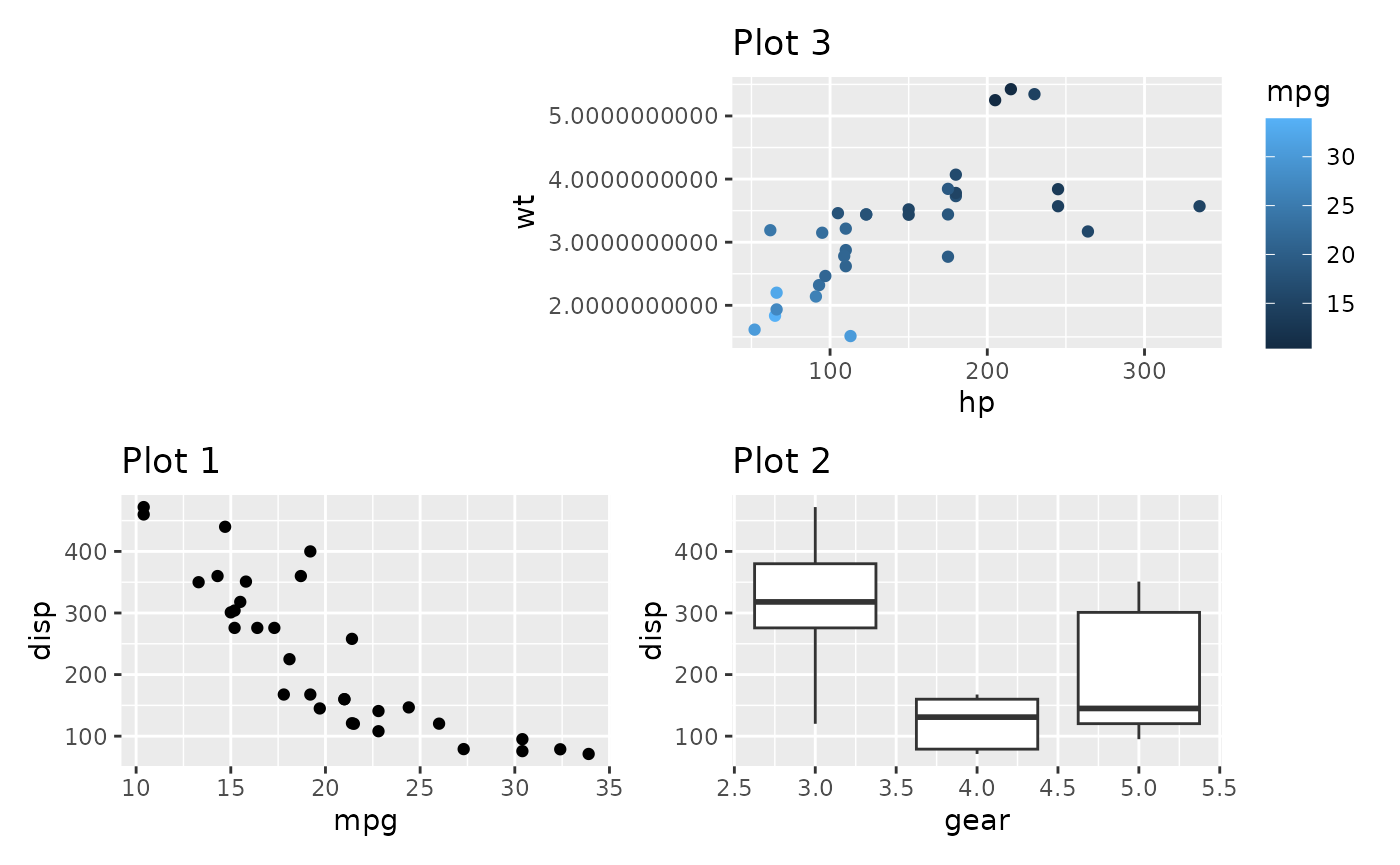## Controlling guides

Plots often have guides to help viewers deduce the aesthetic mapping. When composing plots we need to think about what to do about these. The default behavior is to leave these alone and simply let them follow the plot around. Examples of this can be seen above where the color guide is always positioned beside Plot 3. Such behavior is fine if the purpose is simply to position a bunch of otherwise unrelated plots next to each other. If the purpose of the composition is to create a new tightly coupled visualization, the presence of guides in between the plots can be jarring, though. The plot_layout() function provides a guides argument that controls how guides should be treated. To show how it works, let’s see what happens if we set it to 'collect':

p1 + p2 + p3 + p4 +
plot_layout(guides = 'collect')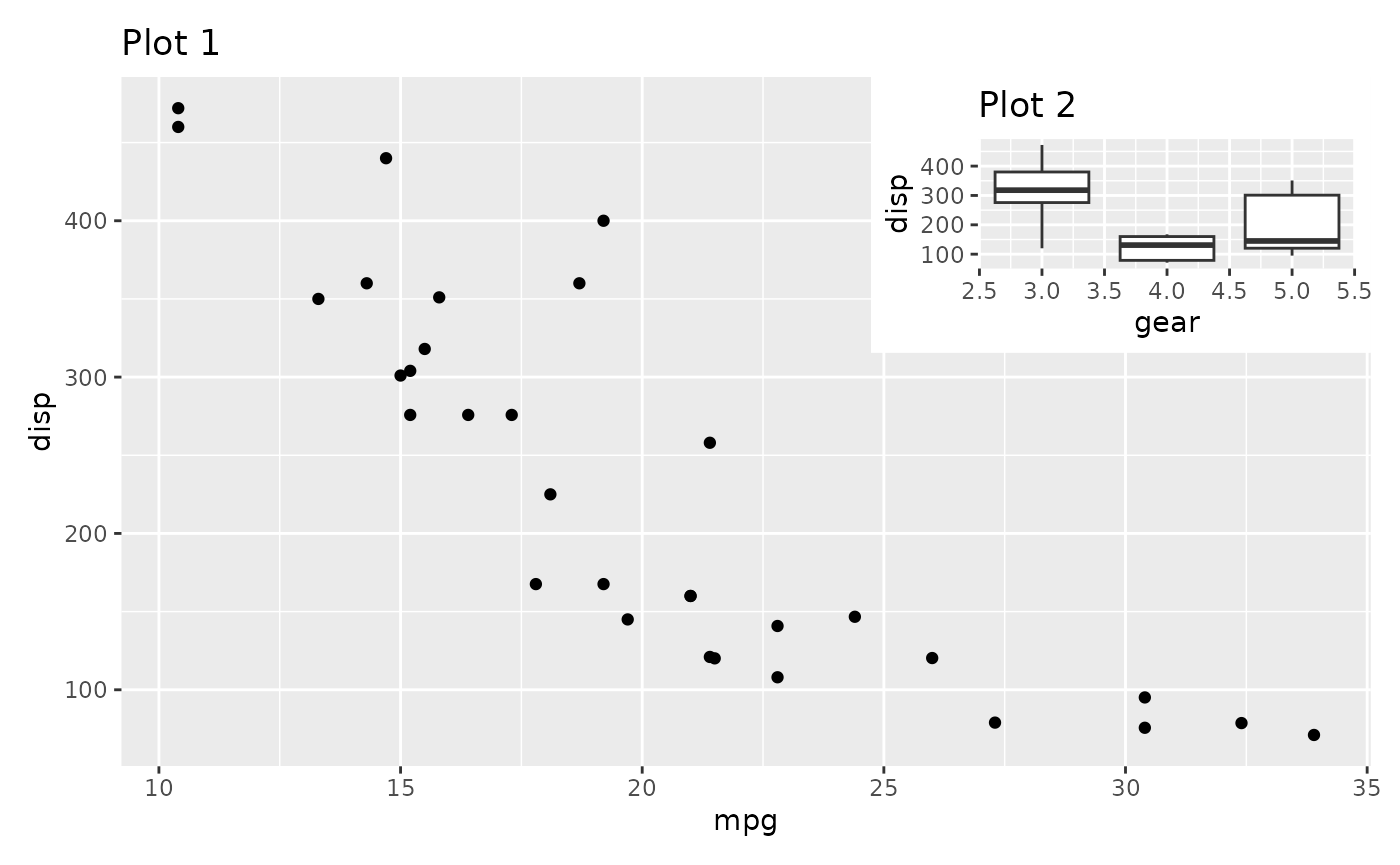We can see that the guide has been hoisted up and placed beside all the plots. The alternative to 'collect' is 'keep', which makes sure that guides are kept next to their plot. The default value is 'auto', which is sort of in between. It will not collect guides, but if the patchwork is nested inside another patchwork and that patchwork collect guides, it is allowed to hoist them up. To see this in effect, compare the two plots:

((p2 / p3 + plot_layout(guides = 'auto')) | p1) + plot_layout(guides = 'collect')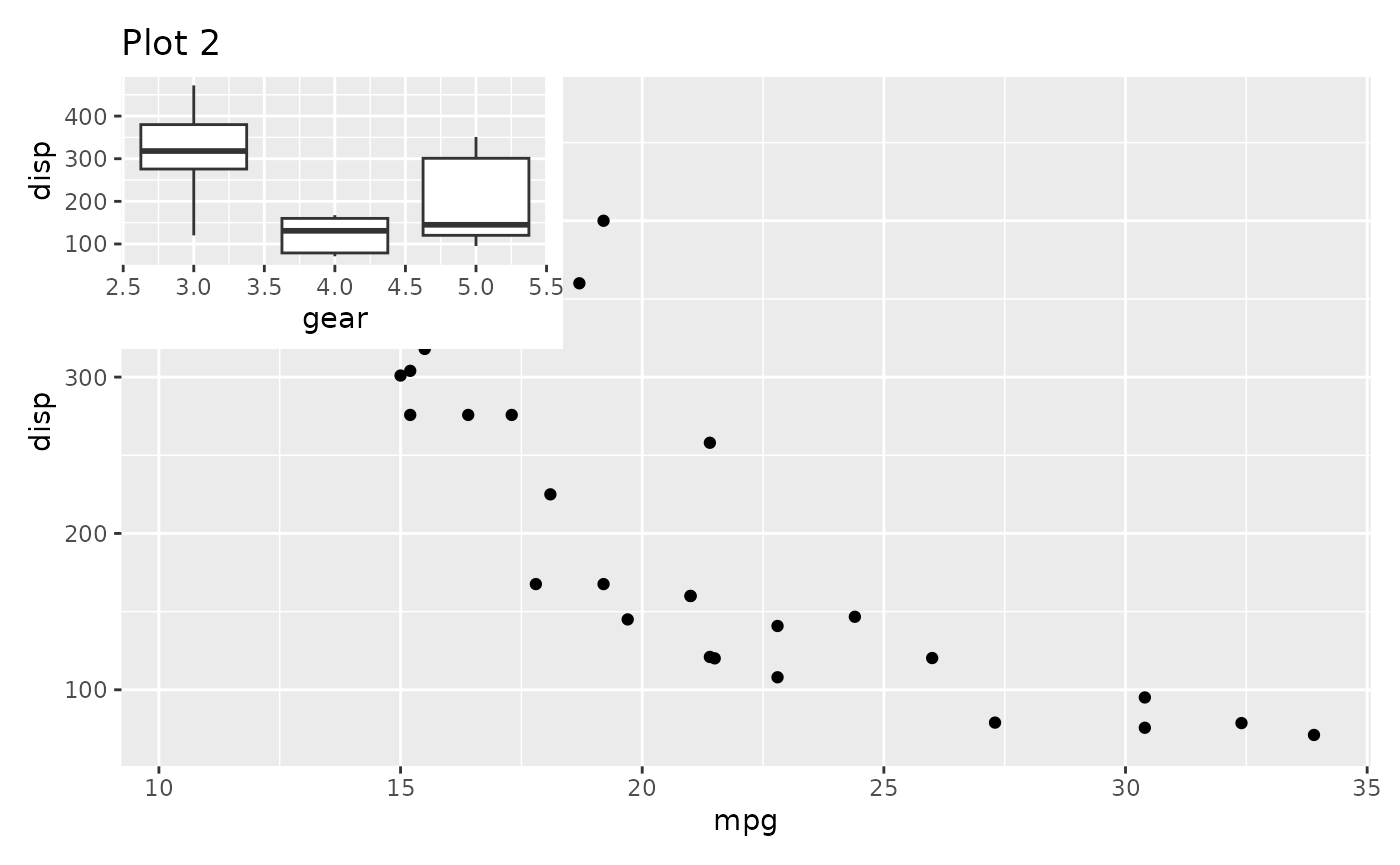((p2 / p3 + plot_layout(guides = 'keep')) | p1) + plot_layout(guides = 'collect')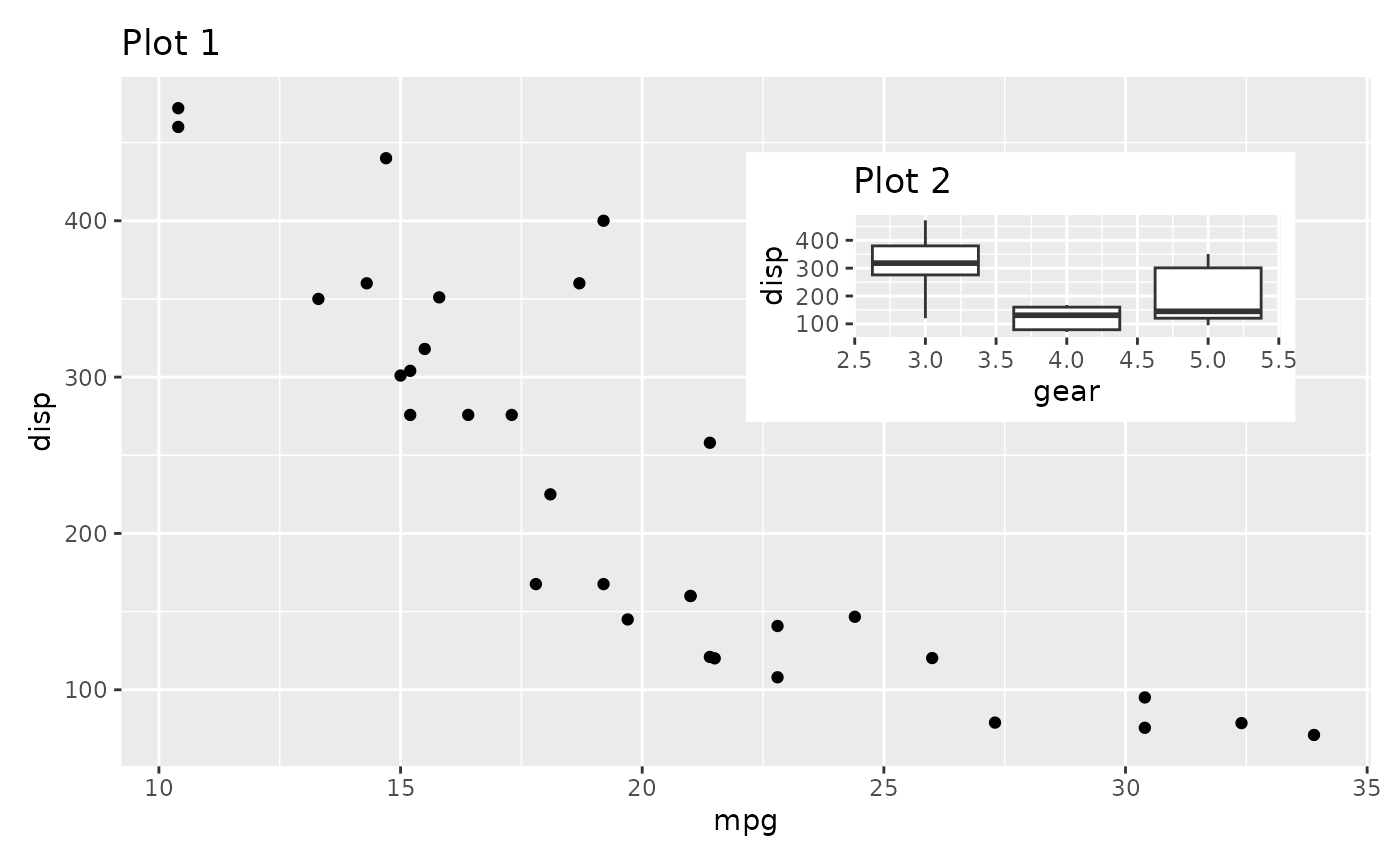The guide collection has another trick up its sleeve. If multiple plots provide the same guide, you don’t want to have it show up multiple times. When collecting guides it will compare them with each other and remove duplicates.

p1a <- ggplot(mtcars) +
geom_point(aes(mpg, disp, colour = mpg, size = wt)) +
ggtitle('Plot 1a')

p1a | (p2 / p3)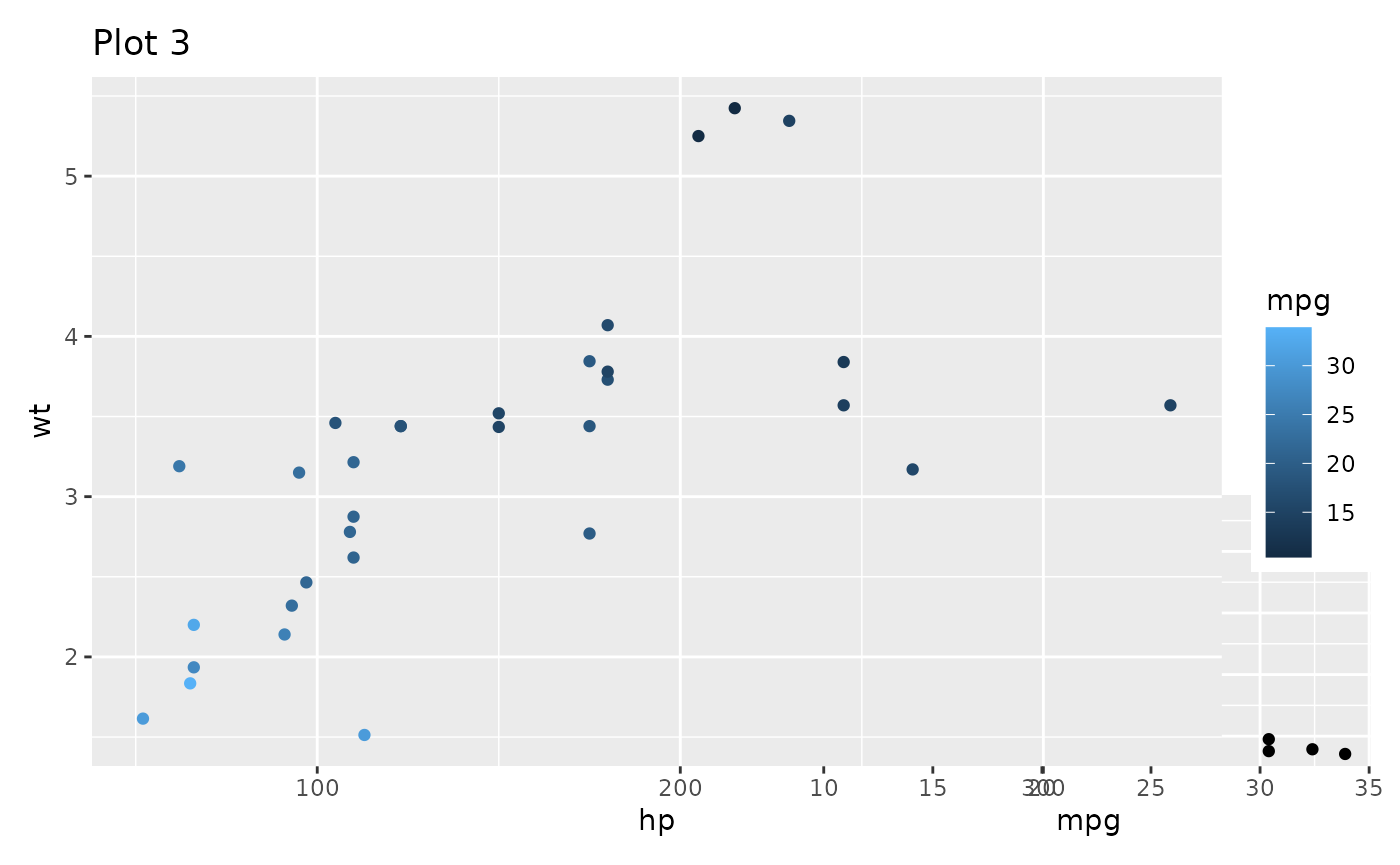(p1a | (p2 / p3)) + plot_layout(guides = 'collect')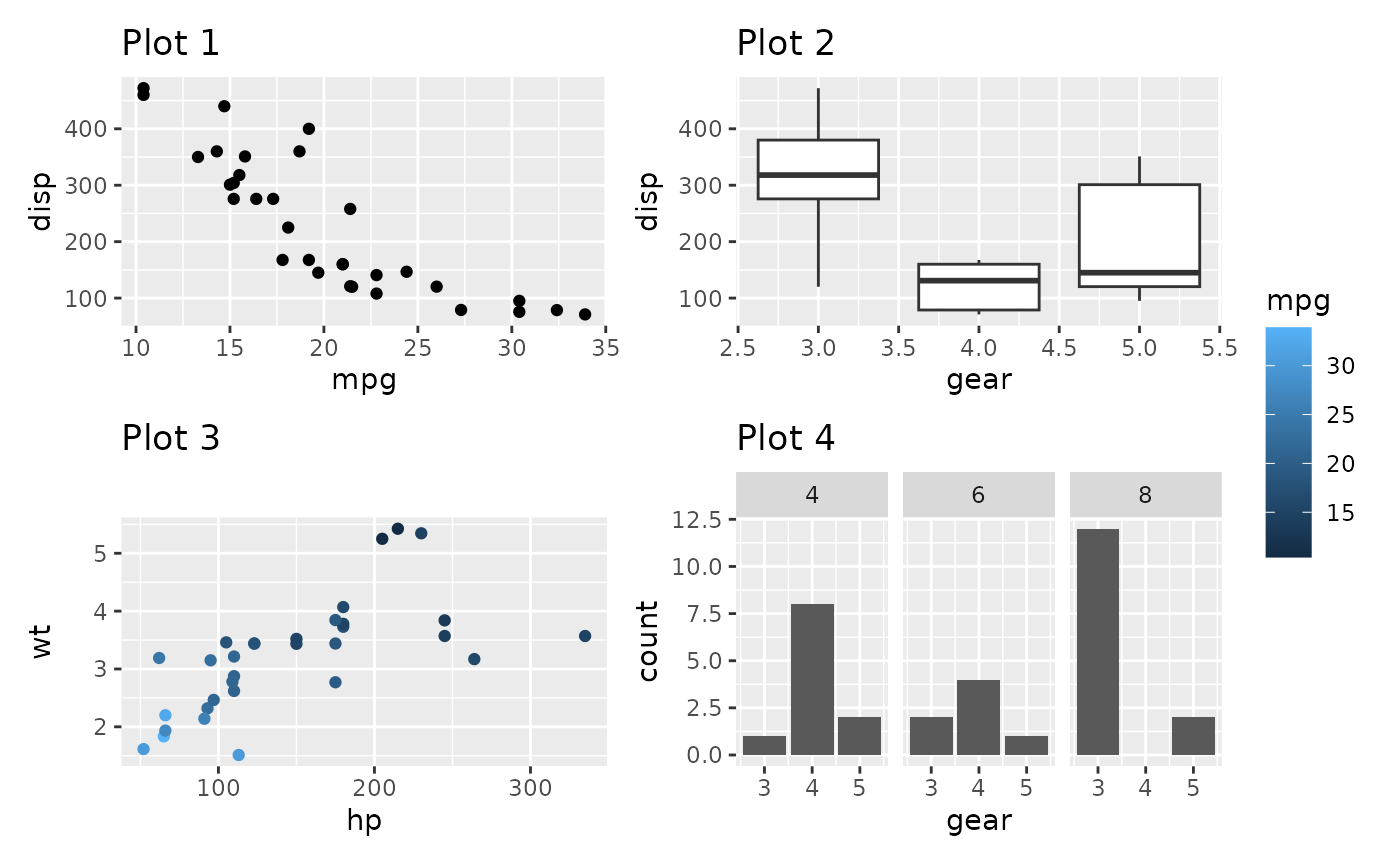Guides are compared by their graphical representation, not by their declarative specification. This means that different theming among plots may mean that two guides showing the same information is not merged.

Now and then you end up with an empty area in you grid. Instead of leaving it empty, you can specify it as a place to put the collected guides, using the guide_area() placeholder. It works much the same as plot_spacer(), and if guides are not collected it will do exactly the same. But if guides are collected they will be placed there instead of where the theme tells it to.

p1 + p2 + p3 + guide_area() +
plot_layout(guides = 'collect')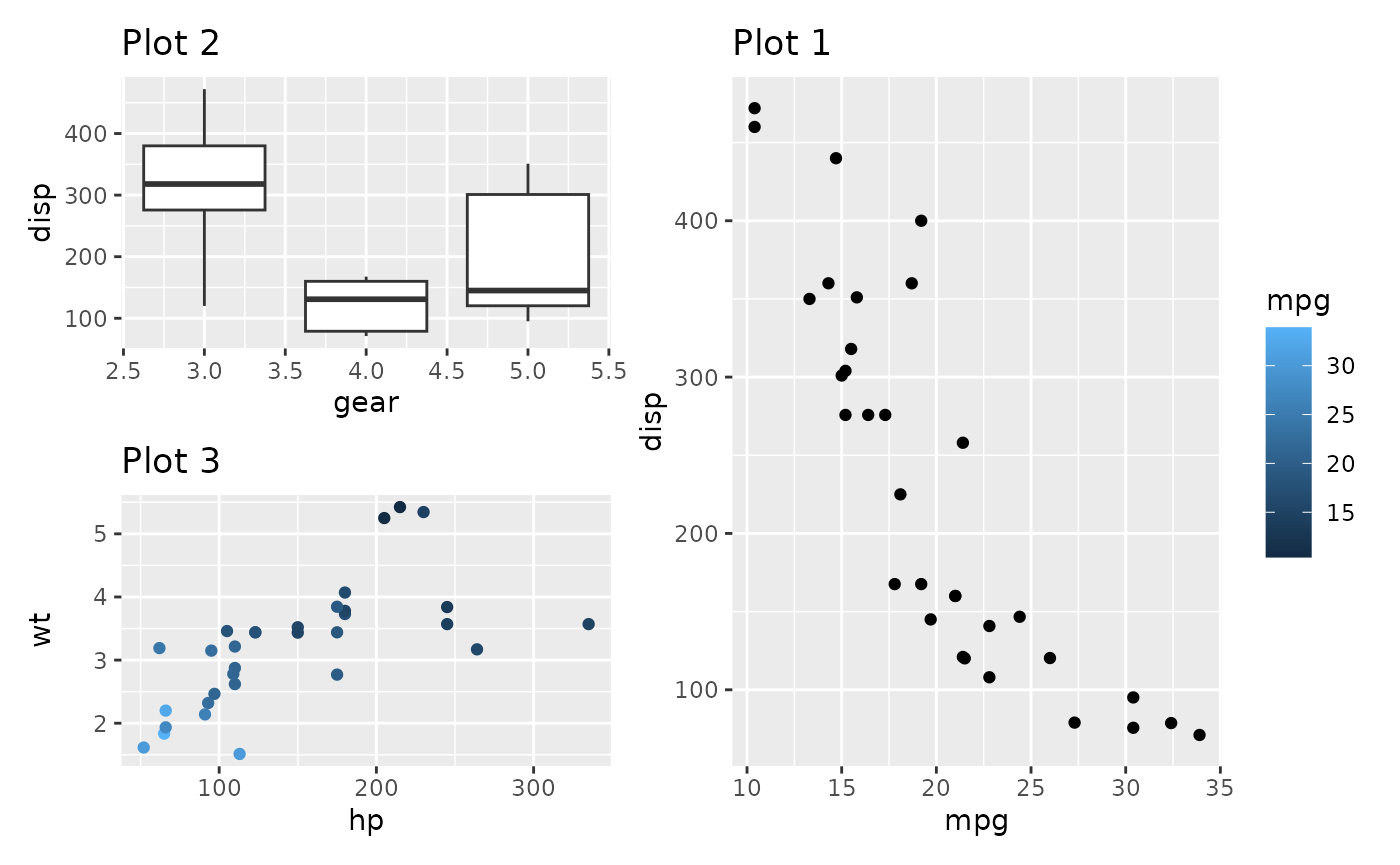Guide areas are only accessible on the same nesting level. If a nested patchwork has a guide area it will not be possible to place guides collected at a higher level there.

## Want more?

This is all for layouts. There is still more to patchwork. Explore the other guides to learn about annotations, special operators and multipage alignment.